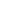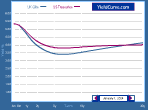Home   What's New   Market Research   Presentations### Case study on bootstrapping zero-coupon and forward rates using RATE software

Mario MelchioriMario Melchiori (Universidad Nacional del Litoral, Santa Fe, Argentina) shows the results of a case study on bootstrapping the yield curve, producing zero-coupon and forward rates from market yields. The spreadsheet shows the results of the exercise, with rates and associates discount factors along the term structure. A supplementary spreadsheet shows the UK yield curve and discount factors... RATE was developed by Rod Pienaar and Moorad Choudhry. RATE will calculate forward rates based on periods that coincide with the zero curve tenors or allows the user to select the length of the forward rate period (quarterly, semi-annual and annual). It is common market practice to calculate each forward period using the curve's tenor points. The calculation is: (1-DF1/DF2)*1/DCF Where: DF1 is the discount factor for the earlier date DF2 is the discount factor for the later date DCF is the day count factor for the tenor (e.g. Act/360). In the case of the case study undertaken by Mario Melchiori and shown on the accompanying spreadsheet, the forward rate for the 3 day to 1 week period for example would be: (1-0.999671/0.999220)*(365/4)=4.11859%

Case study on bootstrapping zero-coupon and forward rates using RATE software (download)

Home   What's New   Market Research   Presentations
RATE yield curve model   Excel Spreadsheets© YieldCurve.comDisclaimerweb design